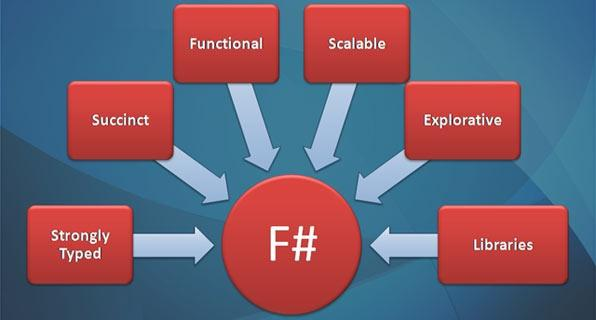JavaScript函数式编程（一）

JavaScript函数式编程（二）

『这些东西有什么卵用？』

``````doSomething()
.then(result => {
// 你可以return一个Promise链！
return fetch('url').then(result => parseBody(result));
})
.then(result => {
// 这里的result是上面那个Promise的终值
})

doSomething()
.then(result => {
// 也可以直接return一个具体的值！
return 123;
})
.then(result => {
// result === 123
})
``````

``````import fs from 'fs';
import _ from 'lodash';

var map = _.curry((f, x) => x.map(f));
var compose = _.flowRight;

return new IO(_ => fs.readFileSync(filename, 'utf-8'));
};

var print = function(x) {
return new IO(_ => {
console.log(x);
return x;
});
}

cat("file")
//=> IO(IO("file的内容"))
``````

``````cat("file").__value().__value();
//=> 读取文件并打印到控制台
``````

``````var join = x => x.join();
IO.prototype.join = function() {
return this.__value ? IO.of(null) : this.__value();
}

// 试试看
var foo = IO.of(IO.of('123'));

foo.join();
//=> IO('123')
``````

``````var cat = compose(join, map(print), readFile);
cat("file").__value();
//=> 读取文件并打印到控制台
``````

join 方法可以把 Functor 拍平（flatten），我们一般把具有这种能力的 Functor 称之为 Monad。

``````var doSomething = compose(join, map(f), join, map(g), join, map(h));
``````

``````var chain = _.curry((f, functor) => functor.chain(f));
IO.prototype.chain = function(f) {
return this.map(f).join();
}

// 现在可以这样调用了
var doSomething = compose(chain(f), chain(g), chain(h));

// 当然，也可以这样

// 写成这样是不是很熟悉呢？
.chain(x => new IO(_ => {
console.log(x);
return x;
}))
.chain(x => new IO(_ => {
// 对x做一些事情，然后返回
}))
``````

（我本来想在下面用 Promise 为例写一些例子，但估计能看到这里的人应该都能熟练地写各种 Promise 链了，所以就不写了0w0）

## 二、函数式编程的应用

1、React

React 现在已经随处可见了，要问它为什么流行，可能有人会说它『性能好』、『酷炫』、『第三方组件丰富』、『新颖』等等，但这些都不是最关键的，最关键是 React 给前端开发带来了全新的理念：函数式和状态机。

``````var Text = props => (
<div style={props.style}>{props.text}</div>
)
``````

``state => virtual DOM => 真实 DOM``

``reducer(currentState, action) => newState``

2、Rxjs

Rxjs 从诞生以来一直都不温不火，但它函数响应式编程（Functional Reactive Programming，FRP）的理念非常先进，虽然或许对于大部分应用环境来说，外部输入事件并不是太频繁，并不需要引入一个如此庞大的 FRP 体系，但我们也可以了解一下它有哪些优秀的特性。

``--- 用户点击了按钮 --> 网络请求成功 --> 用户键盘输入 --> 某个定时事件发生 --> ......``

``````var clicks = Rx.Observable
.fromEvent(document, 'click')
.bufferCount(2)
.subscribe(x => console.log(x)); // 打印出前2次点击事件
``````

``````var inputs = [];
var clicks = Rx.Observable
.fromEvent(document, 'keydown')
.scan((acc, cur) => {
acc.push(cur.keyCode);
var start = acc.length - 12 < 0 ? 0 : acc.length - 12;
return acc.slice(start);
}, inputs)
.filter(x => x.join(',') == [38, 38, 40, 40, 37, 39, 37, 39, 66, 65, 66, 65].join(','))// 上上下下左右左右BABA，这里用了比较奇技淫巧的数组对比方法
.subscribe(x => console.log('!!!!!!ACE!!!!!!'));
``````

3、Cycle.js

Cycle.js 是一个基于 Rxjs 的框架，它是一个彻彻底底的 FRP 理念的框架，和 React 一样支持 virtual DOM、JSX 语法，但现在似乎还没有看到大型的应用经验。

``````import xs from 'xstream';
import {run} from '@cycle/xstream-run';
import {makeDOMDriver} from '@cycle/dom';
import {html} from 'snabbdom-jsx';

function main(sources) {
const sinks = {
DOM: sources.DOM.select('input').events('click')
.map(ev => ev.target.checked)
.startWith(false)
.map(toggled =>
<div>
<input type="checkbox" /> Toggle me
<p>{toggled ? 'ON' : 'off'}</p>
</div>
)
};
return sinks;
}

const drivers = {
DOM: makeDOMDriver('#app')
};

run(main, drivers);
``````